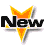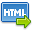Journal :   International Journal of Technology

Volume No. :   4

Issue No. :  1

Year :  2014

Pages :   67-70

ISSN Print :  2231-3907

ISSN Online :  2231-3915Registration

## Goldbach Conjectur and Composite Numbers

 Address: Jagjit Singh Department of Mathematics, Govt. College Chowari District Chamba (H.P.)-176302, India *Corresponding Author
DOI No:

ABSTRACT:
In this paper, the results that every even composite number can be expressed as sum of two odd primes, every odd composite number can be expressed as a sum of an odd prime and a number of the form 2n where n?N and every odd prime number greater than 3 may be expressed as a sum of a pure number and a number of the form 2n where n?N are being presented. Further, for any sufficiently large natural number n, it is presented that every even number up to n can be expressed as a sum of two primes less than n, every even number up to n can be expressed as a sum of two primes less than [?(2/3) n] and every even number up to n can be expressed as a sum of two primes less than[?(3/4) n].
KEYWORDS:
Goldbach Conjectur and Composite Numbers.
Cite:
Dr Jagjit Singh. Goldbach Conjectur and Composite Numbers. Int. J. Tech. 4(1): Jan.-June. 2014; Page 67-70[View HTML][View PDF]

Visitor's No. :   125042